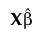##Actual by Predicted Plot
Denote the linear mixed model by E[Y|γ] = Xβ + Zγ. Here β is the vector of fixed effect coefficients and γ is the vector of random effect coefficients. The marginal predictions are the predictions from the fixed effects part of the predictive model, given by.